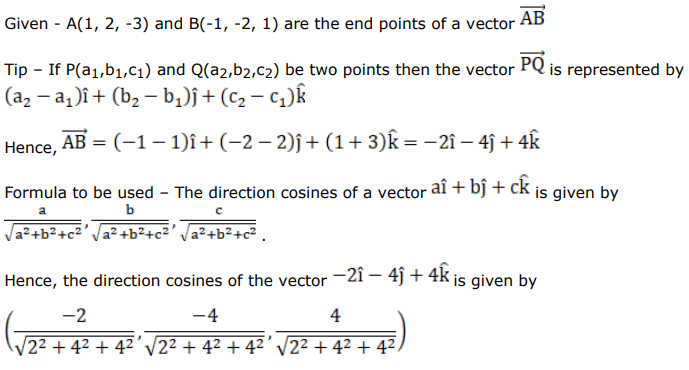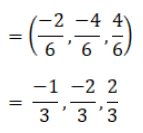# Mark against the correct answer in each of the following:Question:

Mark $(\sqrt{)}$ against the correct answer in each of the following:

If $A(1,2,-3)$ and $B(-1,-2,1)$ are the end points of a vector $\overrightarrow{A B}$ then the direction cosines of $\overrightarrow{\mathrm{AB}}$ are

A. $-2,-4,4$

B. $\frac{-1}{2},-1,1$

C. $\frac{-1}{3}, \frac{-2}{3}, \frac{2}{3}$

D. none of these

Solution: